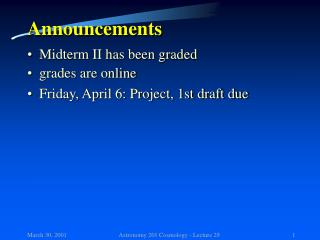Download PresentationAnnouncementsAnnouncements - PowerPoint PPT Presentation

Download PresentationAnnouncements
An Image/Link below is provided (as is) to download presentation

Download Policy: Content on the Website is provided to you AS IS for your information and personal use and may not be sold / licensed / shared on other websites without getting consent from its author. While downloading, if for some reason you are not able to download a presentation, the publisher may have deleted the file from their server.

- - - - - - - - - - - - - - - - - - - - - - - - - - - E N D - - - - - - - - - - - - - - - - - - - - - - - - - - -
Presentation Transcript

1. Announcements • Midterm II has been graded • grades are online • Friday, April 6: Project, 1st draft due Astronomy 201 Cosmology - Lecture 29

2. Final grade boundaries • >90% : A • 75%-90% : B • 60%-75% : C • 45%-60% : D • <45% : E Astronomy 201 Cosmology - Lecture 29

3. Lecture 29The big-bang model II: The cosmological constant: Einstein's greatest blunder or tomorrow's Nobel prize ? Astronomy 201 Cosmology - Lecture 29

4. Let’s switch to general relativity • Friedmann equation • k is the curvature constant Astronomy 201 Cosmology - Lecture 29

5. Let’s switch to general relativity • Friedmann equation • k is the curvature constant • k=0: flat space, forever expanding • k>0: spherical geometry, eventually recollapsing • k<0: hyperbolic geometry, forever expanding Astronomy 201 Cosmology - Lecture 29

6. k>0 k=0 k<0 Astronomy 201 Cosmology - Lecture 29

7. Can we predict the fate of the Universe ? • Friedmann equation: • k=0: Astronomy 201 Cosmology - Lecture 29

8. Can we predict the fate of the Universe ? • If the density  of the Universe •  =crit:flat space, forever expanding •  >crit:spherical geometry, recollapsing •  < crit:hyperbolic geometry, forever expanding • so what is the density of the universe? • We don’t know precisely •  >critvery unlikely • currently favored model: 0.3crit Astronomy 201 Cosmology - Lecture 29

9. How big is crit ? • crit=810-30g/cm3 1 atom per 200 liter • density parameter0 • 0 =1:flat space, forever expanding (open) • 0>1:spherical geometry, recollapsing (closed) • 0<1:hyperbolic geometry, forever expanding • currently favored model:0= 0.3 Astronomy 201 Cosmology - Lecture 29

10. How can we measure 0 ? • Count all the mass we can “see” • tricky, some of the mass may be hidden … • Measure the rate at which the expansion of the universe is slowing down • a more massive universe will slow down faster • Measure the geometry of the universe • is it spherical, hyperbolic or flat ? Astronomy 201 Cosmology - Lecture 29

11. Let’s try to measure the deceleration • Acceleration according to Newton: • deceleration parameter Astronomy 201 Cosmology - Lecture 29

12. So what’s the meaning of q0 ? • deceleration parameter q0 • q0>0.5: deceleration is so strong that eventually the universe stops expanding and starts collapsing • 0<q0<0.5: deceleration is too weak to stop expansion • What’s the difference between q0, 0and k? • k: curvature of the universe • 0: mass content of the universe • q0: kinematics of the universe Astronomy 201 Cosmology - Lecture 29

13. So let’s measure q0 ! • How do we do that? • Measure the rate of expansion at different times, i.e. measure and compare the expansion based on nearby galaxies and based on high redshift galaxies • Gravity is slowing down expansion  expansion rate should be higher at high redshift. Astronomy 201 Cosmology - Lecture 29

14. So let’s measure q0 ! q0 = 0 q0 = 0.5 Data indicates: q0 < 0  Expansion is accelerating fainter more distant Astronomy 201 Cosmology - Lecture 29

15. Science discovery of the year 1998 • The expansion of the universe is accelerating !!! • But gravity is always attractive, so it only can decelerate • Revival of the cosmological constant  Astronomy 201 Cosmology - Lecture 29

16. Friedmann’s equation for >0 • k is the curvature constant • k=0: flat space, flat universe • k>0: spherical geometry, closed universe • k<0: hyperbolic geometry, open universe • k is the curvature constant • k=0: flat space • k>0: spherical geometry • k<0: hyperbolic geometry • but for sufficiently large  a spherically curved universe may expand forever Astronomy 201 Cosmology - Lecture 29

17. Acceleration according to Newton: • deceleration parameterwith Deceleration parameter q for >0 • Acceleration according to Newton: • deceleration parameter Astronomy 201 Cosmology - Lecture 29

18. The fate of the Universe for >0 k=+1 >0 =0 Astronomy 201 Cosmology - Lecture 29

19. Is the fate of the Universe well determined ? • deceleration: • ½0 – > 0: decelerating • ½0 – < 0: accelerating • curvature • 0 + = 1: flat • 0 + < 1: hyperbolic • 0 + > 1: spherical • two equations for two variables  well posed problem Astronomy 201 Cosmology - Lecture 29

20. Cosmology: the quest for three numbers • The Hubble constant H0 • how fast is the universe expanding • The density parameter 0 • how much mass is in the universe • The cosmological constant  • the vacuum energy of the universe • current observational situation: • H0= 65 km/s/Mpc • 0 = 0.3; = 0.7  flat space Astronomy 201 Cosmology - Lecture 29

21. Announcements • Midterm II has been graded • grades are online • Friday, April 6: Project, 1st draft due Astronomy 201 Cosmology - Lecture 29